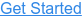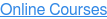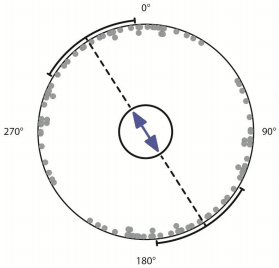Where do you want to go?# Magnetic Fields in QlikView (+ open source QV!)

by Speros Kokenes, on Jan 6, 2014 10:27:02 AM

Recently a zoology study about dog defecation has gone viral. No, seriously.

In an attempt to test dogs' sensitivity to Earth's magnetic field, researchers recorded the urination and defecation of a sample of dogs and plotted the angular position of their bodies during these acts against the magnetic field. The results of the study produced great visualizations like these:Each dot represents the mean angle of a dog across all of it's observations. I believe the dotted line through the middle represents either the mean of all observations and the arcs some sort of confidence interval or magnetic field spread, but I am not certain.

I reproduced the chart in QlikView using radar charts, with the dotted line as the mean and the highlighted arcs as a 95% confidence interval. Random data was used to produce the following:The creation of this chart required several elements:

• loading the inverse of each point; for example, if an event happened at 0 degrees, then a duplicate needed to be created at 360 degrees
• grouping the degrees into bigger buckets in order to plot dots. In this example, I grouped them by units of 5
• creating text labels for only 4 points
• this was accomplished using the a dual function to apply a blank label for every point except the 4 I wanted to show:

dual(if(mixmatch(Degrees,0,90,180,270),Degrees & '°',''),Degrees) as DegreesLabel

• creating a second radar chart in the background for plotting the mean, confidence interval, and complete circle
• the background chart required an isolated set of data that covered all degrees. I autogenerated numbers from 0-360 into an isolated table and then used expressions and variables to add my formatting

Expressions

• Mean dotted line expression

=if(AllDegrees=mod(round(vMean),360) or AllDegrees=mod(round(vMean)+180,360),1)

• Outer circle expression

=1

• Color expression

=if((AllDegrees>=round(vConfidenceIntervalLow) \$(=if(round(vConfidenceIntervalLow)>round(vConfidenceIntervalHigh),'or','and')) AllDegrees<=round(vConfidenceIntervalHigh)) or (AllDegrees>=mod(round(vConfidenceIntervalLow)+180,360) \$(=if(mod(round(vConfidenceIntervalLow)+180,360)>mod(round(vConfidenceIntervalHigh)+180,360),'or','and')) AllDegrees<=mod(round(vConfidenceIntervalHigh)+180,360)),rgb(100,100,100),rgb(200,200,200))

• this expression looks pretty complicated but it is just peforming a simple check to make sure it calculates the ranges correctly. The problem with the range calculations is when they overlap 0 degrees, since the numbers reset. For my implementation I used a logical condition to switch out an "and" or "or" statement, but there may be a better way to solve this problem.

Instead of posting a link to the QVW here, I've decided to give Stefan Walther's idea a try and post the document to GitHub. The script is kept externally from the QVW so that changes can be tracked separately from the front end.

Get it here.

-Speros

Topics:QlikViewVisualization